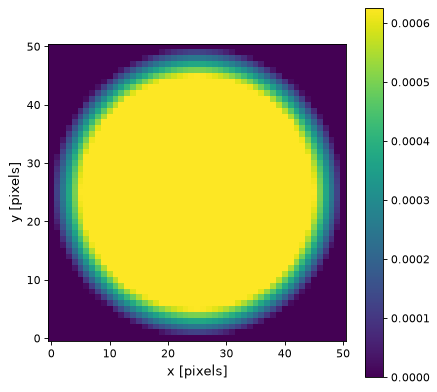# TrapezoidDisk2DKernel¶

class astropy.convolution.TrapezoidDisk2DKernel(radius, slope=1.0, **kwargs)[source]

2D trapezoid kernel.

Parameters: radius : number Width of the filter kernel, defined as the width of the constant part, before it begins to slope down. slope : number Slope of the filter kernel’s tails mode : str, optional One of the following discretization modes: ‘center’ (default) Discretize model by taking the value at the center of the bin. ‘linear_interp’ Discretize model by performing a bilinear interpolation between the values at the corners of the bin. ‘oversample’ Discretize model by taking the average on an oversampled grid. ‘integrate’ Discretize model by integrating the model over the bin. factor : number, optional Factor of oversampling. Default factor = 10.

Examples

Kernel response:

import matplotlib.pyplot as plt
from astropy.convolution import TrapezoidDisk2DKernel
trapezoid_2D_kernel = TrapezoidDisk2DKernel(20, slope=0.2)
plt.imshow(trapezoid_2D_kernel, interpolation='none', origin='lower')
plt.xlabel('x [pixels]')
plt.ylabel('y [pixels]')
plt.colorbar()
plt.show()


()